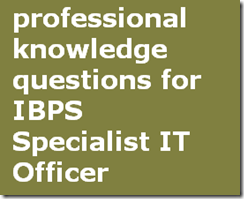## Friday, 22 February 2013

### IBPS IT Specialist Officer professional knowledge Practice -71. 253.45.67.9 is a class---------

[A] D

[B] A

[C] C

[D] E

[E] None of these

(Ans):-D

2. Internal auditors should review data system design before they are

[A] Developed

[B] Implemented

[C] Modified

[D] All the above

[E] None of these

(Ans):-B

3. Artifact based authentication method includes___

[A] Machine readable badges

[B] Electronic smart cards

[C] Both 1 and 2

[D] Vault cards

[E] None of these

(Ans):-C

4. What is the memory from 1K-640K called?

[A] Extended memory

[B] Normal memory

[C] Low memory

[D] Conventional memory

[E] None of these

(Ans):-D

5. I n most WANs, the network contains numerous cables or telephone lines,each one connecting a pair of______

[A] Packet

[B] Star topology

[C] Router

[D] Antennas

[E] None of these

(Ans):-C

6. Which of the following operators in ‘C’ does not associated from the right?

[A] ,

[B] =

[C] +=

[D] ++

[E] None of these

(Ans):-A

7. Which of the following is a variation of frequency division multiplexing (FDM)?

[A] Time Division Multiplexing (TDM)

[B] Pulse Code Multiplexing (PCM)

[C] Wave length Division Multiplexing (WDM)

[D] All the above

[E] None of these

(Ans):-C

8. Race condition occur in which flip-flop

[A] RS flip-flop

[B] JK flip-flop

[C] D flip-flop

[D] T flip-flop

[E] None of these

(Ans):-A

9. The expansion of E-R diagram is:

[A] Entity-Relation diagram

[B] Entity-Relative diagram

[C] Entity-Relation diagram

[D] All the above

[E] None of these

(Ans):-A

10. BNF is a meta-language for

[A] Specifying the syntax of a language

[B] Specifying a context free language

[C] Shell programming

[D] Both 1 and 2

[E] None of these

(Ans):-D

11. What is the language used by most of the DBM’s for helping their users to access data?

[A] High level language

[B] Query language

[C] SQL

[D] 4 GL

[E] None of these

(Ans):B

12. An expression_____

[A] Usually evaluates to a numerical value

[B] Indicate state of the program

[C] May be part of statement

[D] Both 1 and 2

[E] None of these

(Ans):-D

13. HTTP refers to_____

[A] Hyper text transmission protocol

[B] Hyper text transfer protocol

[C] Hyper text tie protocol

[D] Hyper text talent protocol

[E] None of these

(Ans):-B

14. Which language has recently become the difacto standard for interfacing application programs with relational database system?

[A] Oracle

[B] SQL

[C] D base

[D] 4 GL

[E] None of these

(Ans):-B

15. A program that place programs into main memory and prepares them for execution____

[A] Linker

[B] Assembler

[C] Loader

[D] Absolute entity

[E] None of these

(Ans):-C

16. Given √ (224)π = (13)π the value of the radix r is

[A] 10

[B] 8

[C] 6

[D] 5

[E] None of these

(Ans):-D

17. In a Third Normal Form relation, every …. Attribute is non-transitively and fully dependent on the every candidate key Z

[A] Prime

[B] Non-prime

[C] Unique

[D] Network

[E] None of these

(Ans):-B

18. Sockets are used in

[A] Physical layer

[B] Data link layer

[C] Network layer

[D] Transport layer

[E] None of these

(Ans):-B

19. A generalization of the Boyce Code Normal Form to relation schemes which includes the multi valued dependencies is called—

[A] Second normal form

[B] Third normal form

[C] Fourth normal form

[D] Fifth normal form

[E] None of these

(Ans):-C

20. The metal oxide semiconductor (MOS) is a ……….transistor that depends on the flow of only one type of carrier, which may be electrons or holes?

[A] Unipolar

[B] Bipolar

[C] n-polar

[D] s-polar

[E] None of these

(Ans):-A

21. Lexical Analyzer transforms an input streams into---

[A] Regular expression

[B] Sequence of tokens

[C] Binary

[D] Symbols

[E] None of these

(Ans):-B

22. IEEE 802-3

[A] Analog based deterministic

[B] Analog based non deterministic

[C] Digital based deterministic

[D] All the above

[E] None of these

(Ans):-B

23. Depth first search is

[A] Stack

[B] Queue

[C] Link list

[D] Tree search

[E] None of these

(Ans):-A

24. If we traverse a binary search tree, in which manner that we get in ascending manner

[A] In order

[B] Preorder

[C] Post order

[D] Graph

[E] None of these

(Ans):-A

25. Which is the world’s number #1 company for core banking solutions?

[A] Fidelity

[B] Fiserv

[C] Infosys

[D] Oracle

[E] None of these

(Ans):-B

FEEL GOOD THIS MATERIAL COMMENT US UR WORDS

amit said...

Awesome man